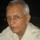Coffee Room
Discuss anything here - everything that you wish to discuss with fellow engineers.
12894 Members
Join this group to post and comment.MURTUZA ZAVERI • Aug 2, 2017

# EQUATION OF SPIRAL

This thing will sound way too complicated. but yet i want to know:

consider that we have a wire made of a very flexible material. the length of wire is L, and the diameter is D.

now if this wire was to be wound in a tight spiral around a rod of diameter say X, then is there any mathematical formula of knowing what the max diameter of the spiral coil will be.?A rigorous analytical solution can be got using integration and the equation for a log spiral. Do not have the time or the inclination to attempt.
A much simpler solution good enough for all practical purposes can be worked out quickly.

Based on data given, the first round will have a length of Pi*(X+D) taking the axis of the wire as reference.
Each successive round will have a length Pi*2*D more than the previous round.

For the given total length of L, let us say n rounds can be made.

Then, the total length can be given as:
L = Pi*[(X+D) + (X+3D) + (X+5D) + …+ (X+(2*n - 1)*D)
= Pi*[n*X + D*(1+3+5….+(2*n - 1))]
= Pi*(n*X + n^2*D) {sum of first n odd numbers = n^2}

Rearranging: D*n^2 + X*n - (L/Pi) = 0

Solve the quadratic for n. Of the two roots only one will be physically feasible.
Since Pi and a variable quantity L are involved, n may not be an integer. The Integral part of n = [n], which means that there would be [n] full turns and a little left over.
The largest diameter measured over the last partial coil portion of the wire will be:
Dia(max.) = X + [n]*D + DIf a rubber yoga or exercise mat is available, this can be verified by winding it tightly over different diameter rods and measuring the diameters. Trial even on one rod will validate the solution.MURTUZA ZAVERI • Aug 3, 2017
THANX A LOT, RAMANI SIRA.V.Ramani
Dia(max.) = X + [n]*D + D
I apologise. There is an error.
The above should be:
Dia(max.) = X + 2*[n]*D + D
Each round adds 2*D to the total diameter.

Share this content on your social channels -